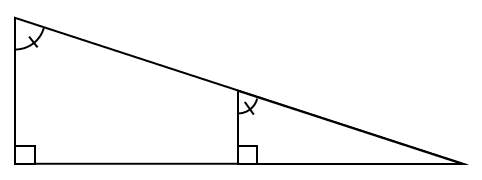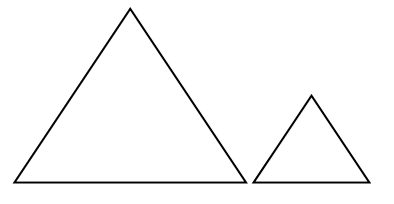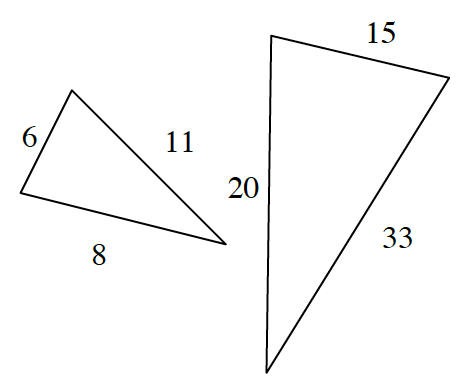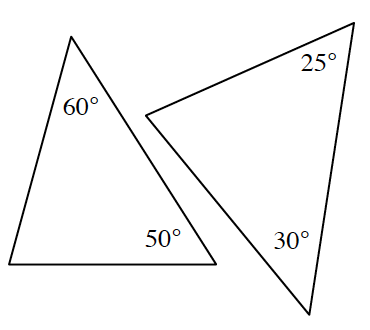### Home > INT2 > Chapter 2 > Lesson 2.3.1 > Problem2-78

2-78.

Decide if each pair of triangles below is similar. If the triangles are similar, justify your conclusion by stating the similarity condition you used. Also, describe a possible sequence of transformations that would carry one onto the other. If the triangles are not similar, explain how you know they are not similar.

1.If two of the corresponding angles are congruent, what do you know about the third pair of angles?

1.equilateral triangles

What are the angle measures of an equilateral triangle?

Triangles are similar by $\text{AA}\sim$ since all angle measures are $60^\circ$. The triangle on the right could be transformed into the triangle on the left by translation and dilation.

1.Write a ratio for each pair of corresponding sides.

1.Find the measures of the unmarked angles.